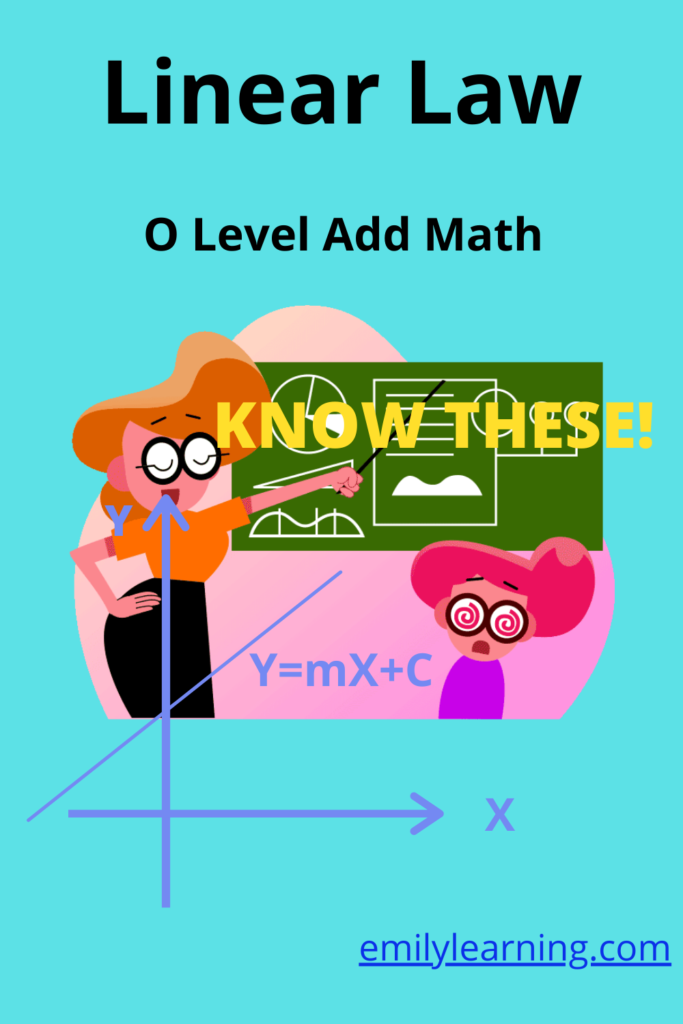# What is tested for linear law tested in O Level Additional MathematicsBasically, in this topic, you are required to convert a relationship between 2 variables to a linear one using linear law rules. You also need to determine the gradient and vertical intercept and from there find the unknown constants.

For instance, if you are given an equation y = axn, how are you going to plot a linear graph for it? Well, you are definitely not going to plot y against x as you won’t get a straight line graph. Instead, you are going to manipulate the equation. For this scenario, you can take logarithm on both sides of the equation:

For  y = axn

by taking log on both sides,

logy = nlogx + log a

If you were to plot log y (vertical axis) against logx (horizontal axis), you will get a straight line with n as the gradient and loga as the vertical intercept.

As you can see, you also need to know coordinate geometry, particularly that involving equation of a straight line is required.

## Want to learn O Level Additional Math on-demand?

Check out our on-demand O Level Additional Math course here.

error: Content is protected !!